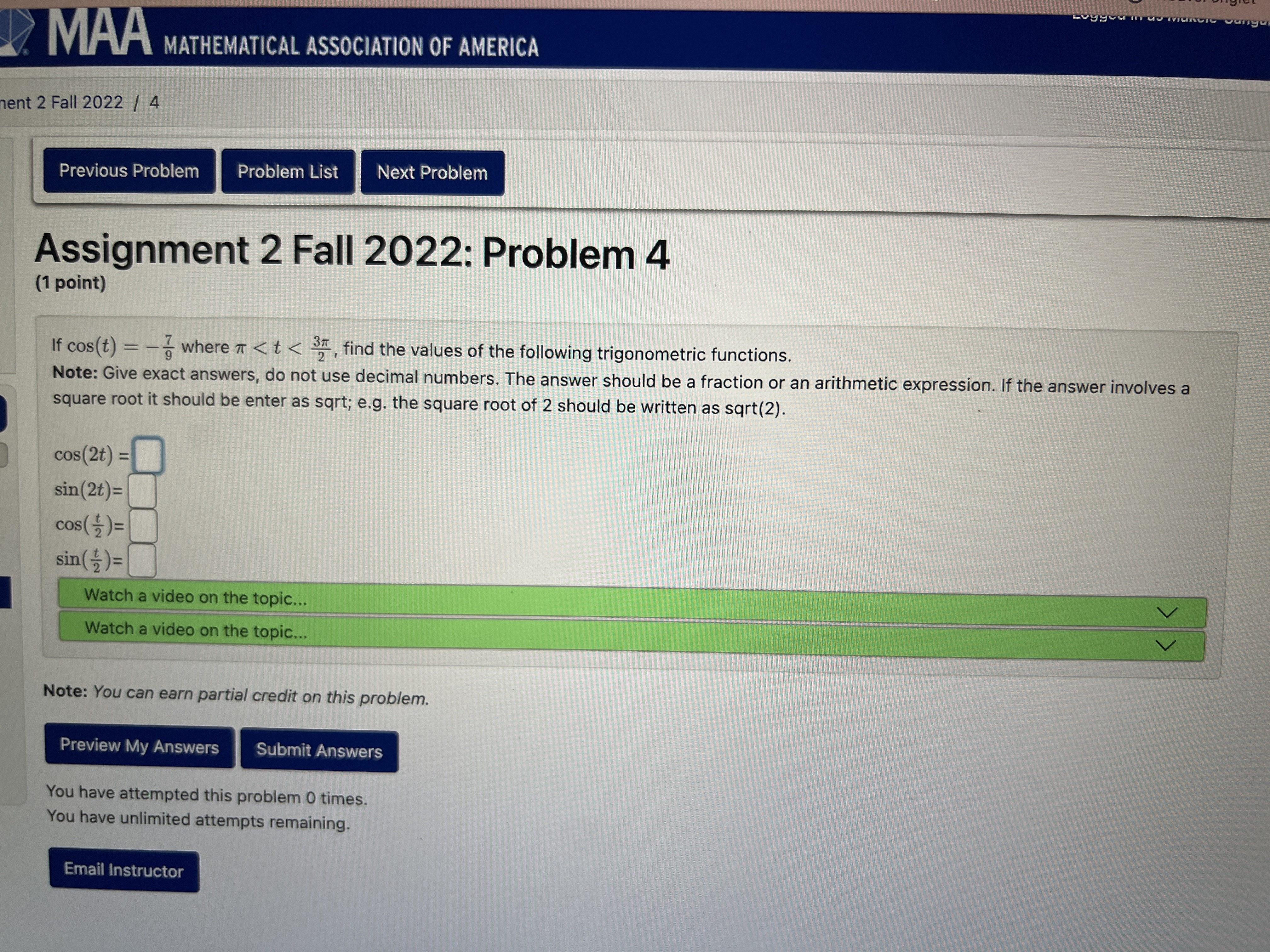# Help Zone

### Student QuestionHello,

I don’t really understand what we should do in this question to find the answers can you explain this to me please?

Mathematics## Explanations (1)

•Explanation from AlloprofExplanation from Alloprof

This Explanation was submitted by a member of the Alloprof team.

Options
Team Alloprof • 4mo. edited September 2022

For the two first questions, I recommend that you use some of the identities for cos(2t) and sin(2t). For example :

$$cos(2t) = 2cos^2t-1$$

$$cos(2t) = 1 - 2sin^2t$$

$$sin(2t) = 2sin(t)cos(t)$$

For the second part (with t/2), I recommend that you use the same formulas as above, but as equations. Specifically, you can consider cos(t) to be equivalent to cos(2•t/2), therefore, for example :

$$-\frac{7}{9} = 2cos^2(\frac{t}{2})-1$$

Don't hesitate to ask for more help if you need it!